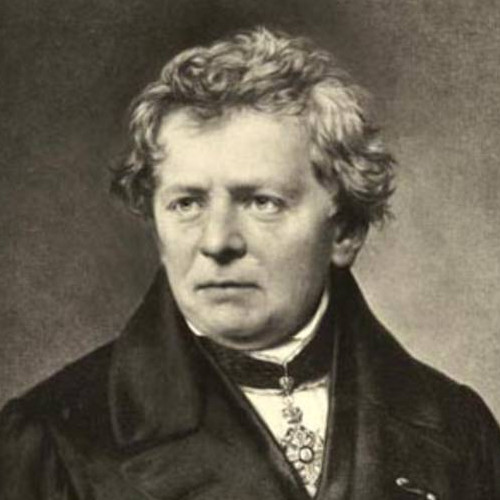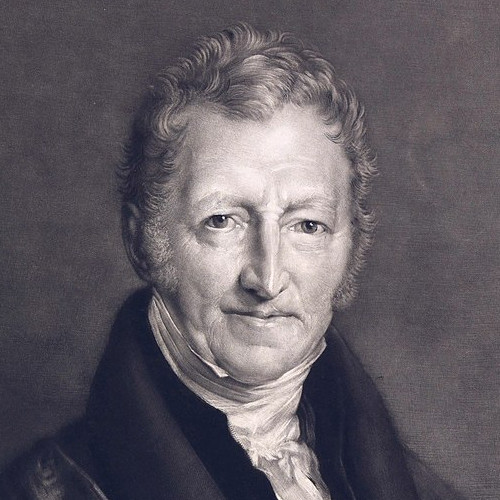# Linearization

The chain rule and the Taylor approximation have lead to linear and quadratic approximations. These approximations are very important in all of sciences as most laws we know in physics, engineering, chemistry or economics are just approximations of more complicated laws. Linear laws are especially appealing. Here are examples:
 Physics: Hooke's law Force is proportional to the displacement Engineering: Ohm's law Potential is proportional to current Chemistry: Ideal gas law Temperature is proportional to pressure Economics: Cobb-Douglas law log(consumption) is proportional to the logs of labor or the log of capital Biology: Malthusian population growth is proportional to population size F = k X V = I R P V = n R T Y = LaKb X' = k XHooke Ohm Clausius Douglas Malthus
These laws are only approximations. In reality, if a spring is extended, there are non-linear effects coming in, due to distortion of the spring for example, when looking at the resistance R related to potential V and current I, also the temperature will play a role (the resistor heats up), the ideal gas law is better described by the van der Vaals law which takes into account that molecules have a volume, the Cobb-Douglas law obviously is a huge simplification as many other parameters are neglected. This law was found by linear data fitting in the log coordinates. The exponential growth law needs to be replaced by the logistic law for larger populations as food resources start to deplete and slow the growth.

By the way if you look at the guys above, you wonder whether it is really fun to discover a law. Cheer up guys! You are famous pop stars of science, for heavens sake!